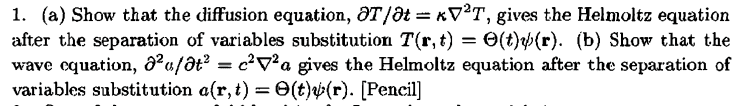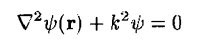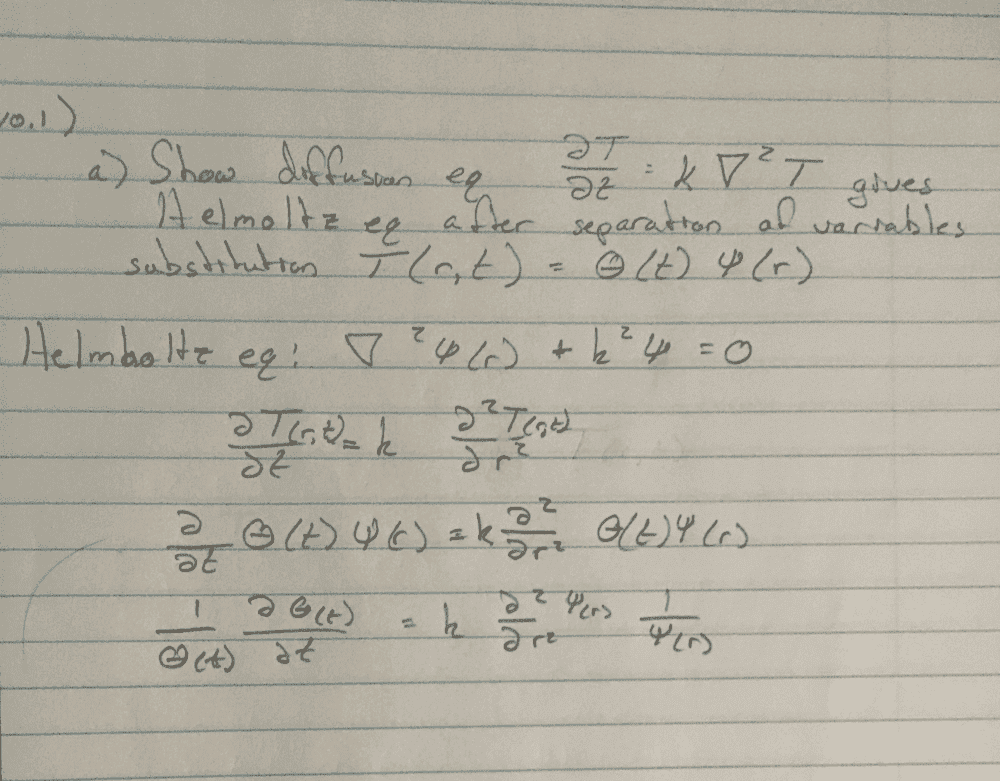# Show that the Diffusion Eqn after substitution gives the Helmholtz Eqn

jkthejetplane
Homework Statement:
Question is shown below. Basically i am wondering if i am over thinking it or am i just plugging it in? I do not see where psi or theta are possible given to do any further work. Same would go for part b.
Relevant Equations:
Helmholtz as defined in my text given below as well
Question:Helmholtz as defined in text:My attempt so far•vanhees71 and Delta2

Homework Helper
2022 Award
So you conclude that each side of your last equation must be equal to some constant $C$, which means that $\psi$ satisfies...

And then you need to justify why $C \leq 0$.

(The constant $k$ which appears in the Helmholtz equation is not necessarily equal to the diffusivity $\kappa$ which appears in the diffusion equation. You need to clearly distinguish between these in your answer.)

•hutchphd, vanhees71 and Delta2
jkthejetplane
So you conclude that each side of your last equation must be equal to some constant $C$, which means that $\psi$ satisfies...

And then you need to justify why $C \leq 0$.

(The constant $k$ which appears in the Helmholtz equation is not necessarily equal to the diffusivity $\kappa$ which appears in the diffusion equation. You need to clearly distinguish between these in your answer.)
Ok so that makes sense as that is how i have started similar problems in the pas that i had boundary conditions for.
I got Θ(t) = Θ(0)eCt and for the other ψ(r) = sin(ψC/$\kappa$) + cos(ψC/$\kappa$)
Then i do the same for Helmholtz eq? OR
Do i set the C/$\kappa$ = $k$? Then plug back in my general solution of ψ(r) and the second derivative of it to the Helmholtz eq hoping to yield 0? Showing that it is only true when $C \leq 0$ ?

Homework Helper
Gold Member
No , i believe its not the easiest path to solve for ##\psi(\mathbf{r})## and then show that this ##\psi## satisfies Helmholtz. It is also wrong that you decide to replace the operator ##\nabla^2## with ##\frac{\partial^2}{\partial r^2}## cause we might be in 2 or 3 dimensions where the ##\nabla^2## operator has different expression. You have shown that $$\kappa\frac{\nabla^2\psi(\mathbf{r})}{\psi(\mathbf{r})}=C$$

From the above equation , how can you manipulate it algebraically to show that it is equivalent to Helmholtz equation ,I repeat again, without solving that equation for ##\psi##, just some algebraic manipulation is all that is needed and replacement of ##\frac{C}{\kappa}## with a proper constant so that we have the Helmholtz equation at the end of the algebraic manipulation.

Last edited:
•vanhees71
jkthejetplane
No , i believe its not the easiest path to solve for ##\psi(\mathbf{r})## and then show that this ##\psi## satisfies Helmholtz. It is also wrong that you decide to replace the operator ##\nabla^2## with ##\frac{\partial^2}{\partial r^2}## cause we might be in 2 or 3 dimensions where the ##\nabla^2## operator has different expression. You have shown that $$\kappa\frac{\nabla^2\psi(\mathbf{r})}{\psi(\mathbf{r})}=C$$

From the above equation , how can you manipulate it algebraically to show that it is equivalent to Helmholtz equation ,I repeat again, without solving that equation for ##\psi##, just some algebraic manipulation is all that is needed and replacement of ##\frac{C}{\kappa}## with a proper constant so that we have the Helmholtz equation at the end of the algebraic manipulation.
So I am just a bit confused still. I need to solve the diff equation for ψ, then just manipulate it to look like Helmholtz?

pai535
So I am just a bit confused still. I need to solve the diff equation for ψ, then just manipulate it to look like Helmholtz?

If you really want to solve a differential equation, you can manipulate it to look like Helmholtz, because it is easy to solve Helmholtz equation (Your teacher told you the formal solution, didn't he?). This also applies to other diff equations that are easy to solve. We always do this when solving Schroedinger equation.

jkthejetplane

If you really want to solve a differential equation, you can manipulate it to look like Helmholtz, because it is easy to solve Helmholtz equation (Your teacher told you the formal solution, didn't he?). This also applies to other diff equations that are easy to solve. We always do this when solving Schroedinger equation.
No he hasn't. This is an upper level class but i had taken a 5 year break from school so i think he assumes we remember everything.

pai535
No he hasn't. This is an upper level class but i had taken a 5 year break from school so i think he assumes we remember everything.

For this question, you only need to give the equation (and maybe don't need to solve it).

For Helmholtz equation, you can solve it by separation of variables.

jkthejetplane
I am not really getting anywhere. Do you have to be super vague on here? I feel like no one gives me direct answers haha. I just want to understand the problem

Homework Helper
Gold Member
No you don't have to solve any equation for ##\psi## (I repeat myself for 3rd time and others have said this as well).
Just algebraic manipulation of this equation $$\kappa\frac{\nabla^2\psi(\mathbf{r})}{\psi(\mathbf{r})}=C$$ and you ll have Helmholtz equation.

Algebraic manipulation means for example that you ll transfer the denominator ##\psi(r)## on the right hand side, then you also may transfer ##\kappa## on the right hand side and then transfer all the right hand side to the left hand side so you ll have equation of "something"=0. That something will look like Helmholtz equation.

pai535
$$\kappa\frac{\nabla^2\psi(\mathbf{r})}{\psi(\mathbf{r})}=C$$

From the above equation , how can you manipulate it algebraically to show that it is equivalent to Helmholtz equation ,I repeat again, without solving that equation for ##\psi##, just some algebraic manipulation is all that is needed and replacement of ##\frac{C}{\kappa}## with a proper constant so that we have the Helmholtz equation at the end of the algebraic manipulation.

If you want to know how to get Helmholtz from diffusion equation, this is what you want. You only need to figure out how you can transform the above equation into the form of Helmholtz.

If you want to solve the Helmholtz equation, I will give a hint in Cartesian coordinates.

Do separation of variables: ##\psi(\vec{r}) = X(x)Y(y)Z(z)##
Then: $$YZ\frac{d^2 X}{dx^2} + ZX\frac{d^2 Y}{dy^2} + XY\frac{d^2 Z}{dz^2} + k^2XYZ = 0.$$
Divide ##XYZ## on both sides: $$\frac{X''}{X}+\frac{Y''}{Y}+\frac{Z''}{Z} + k^2 = 0.$$
You can try to solve it now.

•Delta2
Homework Helper
2022 Award
You can't solve the $\psi$ equation; you don't know what the boundary is or what condition you have to apply there.

You can solve the $\theta$ equation, which will tell you what values of $C$ are physically realistic (because you can rule out values of $C$ which lead to solutions which grow exponentially with time...).

Last edited:
•pai535 and Delta2
$$T(t,\vec{x})=\Theta(t) \psi(\vec{r})$$
•